# 同一工作表完全相同行填充相同颜色

【方法实现】

1、选中数据，在【开始】菜单中选择【条件格式】中的【新建规则】，如下图所示：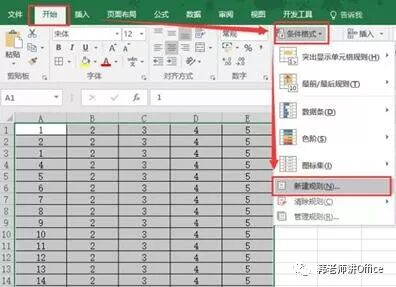2、【新建格式规则】——【使用公式确定要设置格式的单元格】，输入公式：

=SUMPRODUCT((\$A\$1:\$A\$15=\$A1)*(\$B\$1:\$B\$15=\$B1)*(\$C\$1:\$C\$15=\$C1)*(\$D\$1:\$D\$15=\$D1)*(\$E\$1:\$E\$15=\$E1))>1，如下图所示：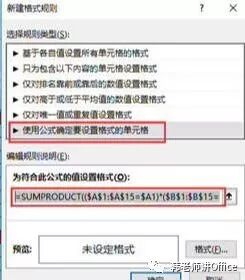3、设置单元格格式填充为你想要的颜色，如下图 所示：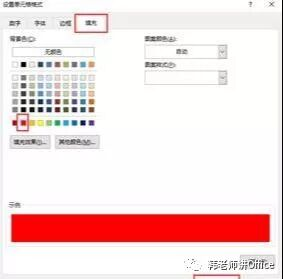【公式解析】

=SUMPRODUCT((\$A\$1:\$A\$15=\$A1)*(\$B\$1:\$B\$15=\$B1)*(\$C\$1:\$C\$15=\$C1)*(\$D\$1:\$D\$15=\$D1)*(\$E\$1:\$E\$15=\$E1))>1

# 不同工作表完全相同行填充相同颜色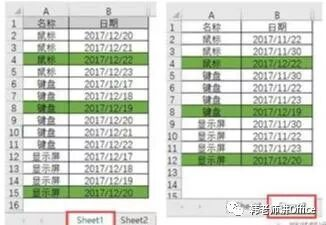【方法实现】

1、选中数据sheet1的A2：B15区域，在【开始】菜单中选择【条件格式】中的【新建规则】，如下图 所示：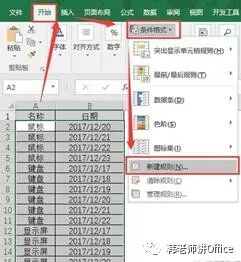2、【新建格式规则】——【使用公式确定要设置格式的单元格】，输入公式：

=SUMPRODUCT((Sheet2!\$A\$2:\$A\$12=\$A2)*(Sheet2!\$B\$2:\$B\$12=\$B2))>=1：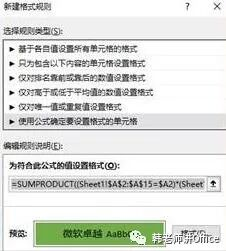3、设置单元格格式填充为你想要的颜色。

=SUMPRODUCT((Sheet1!\$A\$2:\$A\$15=\$A2)*(Sheet1!\$B\$2:\$B\$15=\$B2))>=1，如下图所示：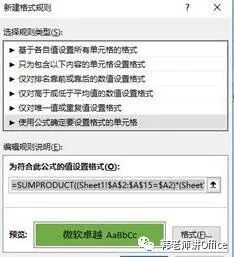sheet1中：

=SUMPRODUCT((Sheet2!\$A\$2:\$A\$12=\$A2)*(Sheet2!\$B\$2:\$B\$12=\$B2)*(Sheet2!\$C\$2:\$C\$12=\$C2))>=1

sheet2中：

=SUMPRODUCT((Sheet1!\$A\$2:\$A\$15=\$A2)*(Sheet1!\$B\$2:\$B\$15=\$B2)*(Sheet1!\$C\$2:\$C\$15=\$C2))>=1